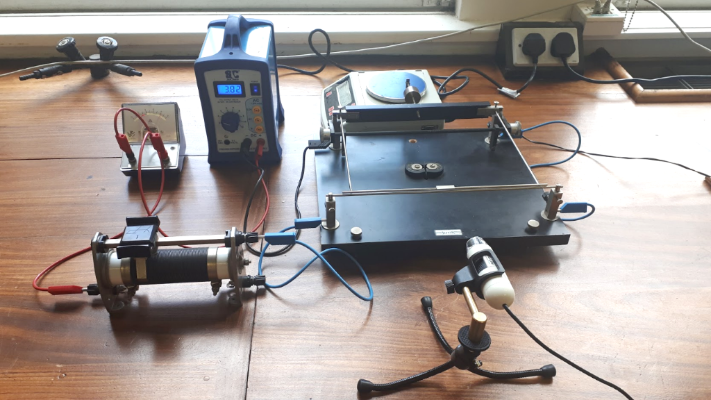# Current Balance

When electrity runs through a wire it is said that an electric current runs through the wire. This current then produces a magnetic field around the wire. The direction of the magnetic field is dependant on the direction the current is ravelling. A fantastic animation of this can be seen here. If two wires have current travelling through them but in opposite directions they would produce opposing magnetic fields. The opposing magentic fields repel each other.

The current balance is used to measure the force of repulsion between identical, oppositely directed, currents in parallel conductors.  This shows a direct link between the mechanical forces of Newton and the electromagnetic forces of Ampere and Maxwell.

 Close up Pic Gif of movable wire

This current balance apparatus has been used for decades in the Department of Physics, UCC and in other college worldwide. As can be seen in the image above there is one fixed wire and one movable wire. The movable wire has balances on the apparatus on two knife edges. There is a counterweight on the opposite side of the wire so that the movable wire can be balanced exactly above the fixed wire, but not touching.

 Close up of knife edges Close up of wires almost touching

When the apparatus is wired up correctly it is all one circuit but the current passes through the wires in opposite directions. When the power is turned on the wires repel. However it can be hard to measure the force on the movable wire this way. In the modern version of this experiment a weighing scales is used. The weighing scales is placed under the counterbalance of the movable wire. It is raised up so it is just touching the counterbalance but there is no weight on it. When the current balance is turned on the moveable wire can no longer move away from the fixed wire and as such it presses down on the weighing scales.

 Gif of repulsionAs talked about in the first experiment the weighing scales uses the force of gravity on an object to calculate the mass of the object. As such known F=ma and that a = 9.81 m/s^2 (acceleration due to gravity) and the mass is the mass reading from the weighing scales then the force of the repulsion can be calculated.

## Department of Physics

Roinn na Fisice

Room 213 (Physics Office), 2nd floor, Kane Science Building, University College Cork, Ireland.,

Top